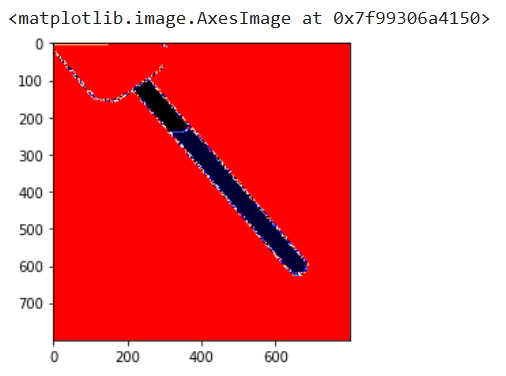# Controling the segmantation prediction better

I have the `segmentation.deeplabv3_resnet50` under my test. It’s working bad at the moment.

As you can see this is the original image normalizedAnd this is the ground truth with just 3 classes (background - red, vessel - black, and filled - blue).I just don’t have the experience training this model. Exactly:

1. What should be the final prediction? Single channel image or 3 channel image? My ground truth is just a single channel image 1x800x800 and it has 3 values inside: background 0, vessel 1 and filled 2. So should I create 3 channel image from 1x800x800?

Note: I used `cmap="flag"` in matplotlib to show the ground truth.

1. Is it possible to prohibit the prediction images to have other values than 0, 1, and 2?

Currently my prediction is 3 channels:

``````net.eval()
pred=net(imtest)['out']
pred.squeeze(0).shape
``````

torch.Size([3, 800, 800])

When I created the model I had 2 options.

``````net = torchvision.models.segmentation.deeplabv3_resnet50(num_classes=3)
``````

and 2nd:

``````net = torchvision.models.segmentation.deeplabv3_resnet50(pretrained=True) # Load net
net.classifier = torch.nn.Conv2d(256, 3, kernel_size=(1, 1), stride=(1, 1)) # Change final layer to 3 classes
``````

but I selected the 2nd option.

Also you may saw values other than 0, 1, 2 on the edges.The original target PNG images have just 0,1,2 values inside, after transforms resize I think the default resizer produces that edge values don’t have the 0,1 and 2 any more instead they are somewhere in between because of interpolation.

1. Any ideas I suppress that.
1. The output for a multi-class segmentation use case would usually have the shape `[batch_size, nb_classes, height, width]` where `num_classes=3` in your use case.

2. Each channel of the prediction contains the logit values for the corresponding class. Using `torch.argmax(output, dim=1)` will return the predicted class indices in the range `[0, 2]`.

3. Yes, I think you are correct and these values are created from e.g. a linear interpolation during resizing. Use the nearest neighbor interpolation technique for any `Resize` transformation and these values should disappear.

1 Like